# Integral

(Redirected from Integrate)
Jump to: navigation, searchThis article/section deals with mathematical concepts appropriate for late high school or early college.

An integral is a mathematical construction used in calculus to represent the area of a region in a plane bounded by the graph of a function in one real variable. Definite integrals use the following notation: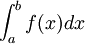where a and b represent the lower and upper bounds of the interval being integrated over, f(x) represents the function being integrated (the integrand), and dx represents a dummy variable given various definitions, depending on the context of the integral.

Indefinite integrals use the notation## Integration

### Definition

If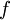is a function of bounded variation, then the Riemann integral ofis defined as the limit of a Riemann sum: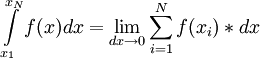.

The fact thatis of bounded variation implies that the Riemann sum above converges and is independent of the choices of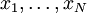. For integration of measurable functions which may not have bounded variation, the Lebesgue integral must be used. It is easy to see thatis the area under the curve f(x) between the vertical line x=a and the vertical line x=b. a and b are called the limits of the integral, and f(x) is called the integrand. This type of integral is referred to as a definite integral, or an integral between definite limits.

Indefinite integrals are related to definite integrals by the second part of the Fundamental Theorem of Calculus.

### Applications

The first part of the Fundamental Theorem of Calculus states that integration is the reverse function of differentiation. Thus, if, then.

Note that, because the derivative of any constant function is 0,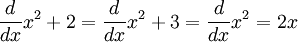. Therefore,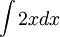does not simply equal x2, but rather x2 + C for any constant real number C. The above-mentioned functions are the family of antiderivatives for 2x.

The concept of integration can be extended to functions in more than one real variable, as well as functions defined over the complex numbers.

Integration has many physical applications. The indefinite integral of an acceleration function with respect to time gives the velocity function defined to within a constant, while the definite integral of an acceleration function with respect to time gives the change in velocity between the upper and lower limits of integration. Likewise, the indefinite integral of a time function of velocity with respect to time gives the position function defined to within a constant, and the definite integral of this velocity function will give the change in position between the two limits of integration.

### Methods of Integration

For a more detailed treatment, see Methods of integration.
There are many different ways to integrate functions. Sometimes, when it is impossible to directly integrate a function, an approximation is used, such as the Riemann integral. Or, there may be a process to integrate the function using a rule, such as with Integration by parts.

## Types of Integrals

There are several types of integrals. Definite integrals are integrals that are evaluated over limits of integration. Indefinite integrals are not evaluated over limits of integration. Evaluating an indefinite integral yields the antiderivative of the integrand plus a constant of integration.

A third type - an improper integral - is an integral in which one of the limits of integration is infinity. Evaluating an improper integral requires taking the limit of the definite integral as the appropriate limit of integration approaches infinity.

A fourth type of integral is known as the Lebesgue integral. The Lebesgue integral is a generalization of the Riemann integral that allows traditionally and non-integrable functions such as the indicator function on the rational numbers to be integrated.

## Properties of integrals

Integration has the following properties

### Additive Distribution

If an integral contains two functions added together, it may be rewritten as two separate integrals, each containing one function.### Constant Multiplicative Distribution

If an integral contains a function multiplied by a constant, it may be rewritten as the constant times the integral of the function.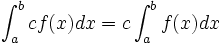### Area

Any integral that contains the same upper and lower bounds is equal to zero.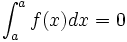### Separation

An integral may be rewritten as the sum of two integrals with adjacent bounds.,### Additive Inversion

Any integral is equal to the additive inverse of the same integral with reversed upper and lower bounds.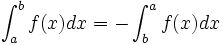## Antiderivative vs Integration

There are important differences between the anti-derivative and integration. An anti-derivative of a function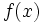is a functionsuch that,The integral of a function can be evaluated using its antiderivative,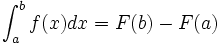This works for the kind of functions encountered in late high school and early university mathematics. It is, however, an incomplete method. For example, one cannot write the anti-derivative ofin terms of familiar functions (such as trigonometric functions, exponentials, and logarithms) and function operations.

## Riemann integral

For a more detailed treatment, see Riemann Integral.
As a geometric interpretation of the integral of the area under a curve, the Riemann integral consists of dividing the area under the curve of the function into slices. The domain of the function is partioned into N segments of widthThe height of the segment is dependent on which side of the rectangle is taken. The lower sum takes the lower side of the rectangle, the upper sum the higher side of the rectangle. In the limit of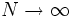these two series become the integral. If they approach the same value then the integral exists, otherwise it is undefined.

### Simpson's Rule

For a more detailed treatment, see Simpson's rule.
Simpson's Rule is an extension of the Riemann integral. Instead of using shapes such as rectangles or trapezoids, Simpson's Rule allows for the use of parabolas or other higher order polynomials to approximate integrals.

## Multiple integralsA double integral gives the volume under a function over a given area - here, the area under the function (at top) within a square.

Multiple integrals are integrals extended to higher dimensions. Just like a definite integral gives the area under a 2-dimensional function, a double integral gives the volume under a three-dimensional function, and a triple integral gives the four-dimensional volume under a four-dimensional function. An ordinary integral is integrated over a single variable, such as x; similarly, a double integral is integrated over a two-dimensional area, usually written A; and a triple integral is integrated over a three-dimensional volume, usually written V.

A double integral:A triple integral: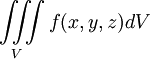Fubini's Theorem states that, for double integrals,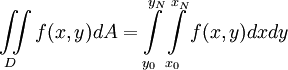and for triple integrals,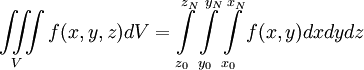These functions can be integrated with respect to the variables x, y, and z in any order. For example, the double integral above is also equivalent to.

## Lebesgue Integral

The Lebesgue integral is usually introduced in late university or early postgraduate mathematics. It is naively described as rotating the Reimann integral, in that it is the range instead of the domain that is partitioned. An understanding of measure theory is required to understand this technique.

The Lebesgue integral is defined for every function for which the Riemann integral is defined, as well as for an even larger class of functions: this possibility of integrating functions which are not Riemann integrable is a large part of the motivation for the Lebesgue theory. A typical example of a function which is Lebesgue integrable but not Riemann integrable is the characteristic function of the rationals,, defined by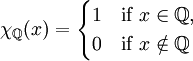.

Because the rationals are only countable, this function is zero "almost everywhere": there are far more irrationals than rationals, and the function isat all of these. Thus we would expect that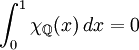Unfortunately, this function has so many discontinuities that its Riemann integral is not defined. However, if we use the Lebesgue integral instead, the function is integrable as hoped, and has the expected value.